### Common Base configuration: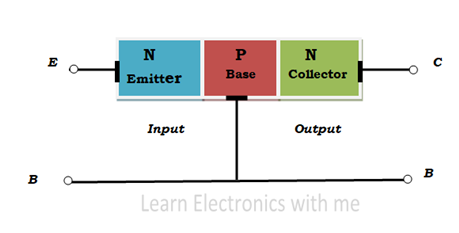Common Base configuration

Two terminals are needed for input and two terminals are needed for output, so one terminal is taken as common for both input and output.  Based on the terminal which is taken as common there are three types of configurations. They are

• Common base configuration
• Common emitter configuration
• Common collector configuration

In common base configuration base terminal taken as common for both input and output. Input is applied between emitter and base terminal and output is taken between collector and base terminal.

Common emitter configuration and Common collector configuration are widely used and common base configuration is the least used.

### Circuit diagram of Common Base NPN and PNP transistor:

In the Common base circuit for NPN and PNP the input is given between emitter and base terminals and output is taken from collector and base terminals. The input voltage is denoted as VBE and the output voltage is denoted as VCE. In all the configuration the base emitter junction is always forward biased and the collector base junction is reverse biased.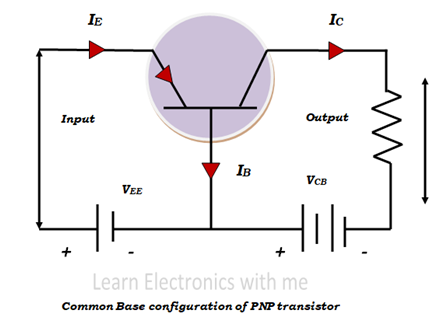Common Base configuration of PNP transistor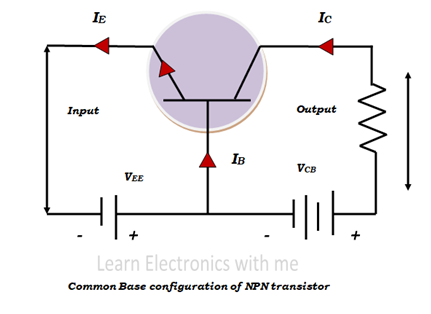Common Base configuration of NPN transistor

In the common base configuration of NPN circuit emitter is N type base is of P type and collector is of N type. The emitter base terminals are forward biased so the majority charge carriers in the emitter that is the electrons gets repelled by the negative applied voltage and in the same way the majority charge carriers in the base that is the holes gets repelled by the positive applied voltage.

When free electrons from emitter move to the base the free electrons and the free holes combine with each other but since the base is very thin only some free electrons gets combined with the holes and the majority of the electrons are attracted towards the collector because of the positive terminal voltage connected to the collector. Thus the current flow through the output terminal.

Thus the emitter current is the sum of the base current and the collector current.

IE = IB+IC

In common base configuration, the input impedance is low and the output impedance is high and the overall power gain is low when compared with other configuration.

### Input characteristics: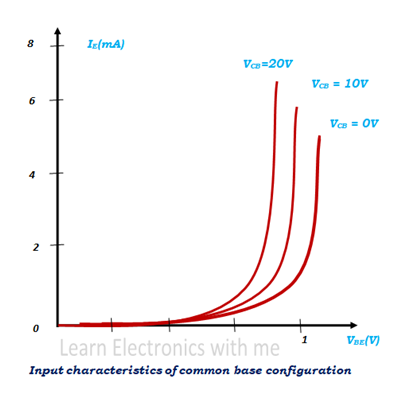Input characteristics of Common Base configuration

Input characteristics are the relationship between the input current and input voltage with constant output voltage. In common base configuration input current is emitter current IE and the input voltage is base emitter voltage VBE. The curve is plotted between IE and VBE keeping VCB as constant.

The VBE is increased keeping VCB constant, initially at zero and the input current IE is noted, similarly the VCB value is increased and kept constant and VBE is increased and the input current IE is noted.

Input side is forward biased so the input resistance is small so for a small increase in VBE there is rapid increase in the emitter current IE. As the output voltage VCB is increased the width of the depletion layer between emitter base decreases and the cut in voltage is reduced so the curve drifts to the left side.

### Output characteristics: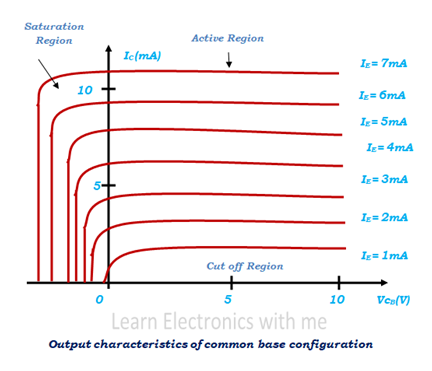Output characteristics of common base configuration

Output characteristics are the relationship between output current IC and output voltage VCB keeping input current IE constant. When the input current IE is zero it is in cut off region. In saturation region both emitter base junction and collector base junction are forward biased.

In active region IE is gradually increased and kept constant and output voltage VCB is increased further and the output current IC almost remains constant. So in active region curve is almost flat. Output voltage causes only a very little change in output current.